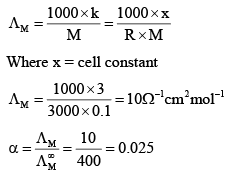Courses

# Test: Conductance - 2

## 30 Questions MCQ Test Physical Chemistry | Test: Conductance - 2

Description
This mock test of Test: Conductance - 2 for Chemistry helps you for every Chemistry entrance exam. This contains 30 Multiple Choice Questions for Chemistry Test: Conductance - 2 (mcq) to study with solutions a complete question bank. The solved questions answers in this Test: Conductance - 2 quiz give you a good mix of easy questions and tough questions. Chemistry students definitely take this Test: Conductance - 2 exercise for a better result in the exam. You can find other Test: Conductance - 2 extra questions, long questions & short questions for Chemistry on EduRev as well by searching above.
*Multiple options can be correct
QUESTION: 1

### Which of the following options are correct:

Solution:
*Multiple options can be correct
QUESTION: 2

### The correct expressions between conc. mobility and molar conductivity are:

Solution:
*Multiple options can be correct
QUESTION: 3

### Which of the following are correct:

Solution:
QUESTION: 4

Which of the following expressions are correct:

Solution:

Explanation : Al2(SO4)3 --------> 2A(Al3+) + 3SO42-

We can calculate the equivalent conductance only for ions, so the equivalent conductance at infinite dilution,

∧∞eq = ∧oAl3+ + ∧oSO42-

*Multiple options can be correct
QUESTION: 5

Which of the following plots are correct:

Solution:
*Multiple options can be correct
QUESTION: 6

The correct expressions of molar conductivity are:

Solution:
*Multiple options can be correct
QUESTION: 7

The correct expressions for dissociation constant of weak acid are:

Solution:
*Multiple options can be correct
QUESTION: 8

The correct expressions which explain the Debye-Huckel-Onsager Theory for strong electrolytes are

Solution:
QUESTION: 9

The correct order of molar conductivities at infinite dilution are:

Solution:

Explanation :  Li+ would be the smallest because it has only 2 electrons and therefore the least electron- electron repulsion.

K+ is larger than Na+ because the ionic radius increases in a particular group on moving from top to bottom due to increase in the principle energy shell though the number or electrons in the valence shell remain the same.

The conductivity of an ion depends mainly on its mobility. That is how fast it moves in an ionic solution. H+ being the smallest ion moves fast. So it has the highest conductivity.

*Multiple options can be correct
QUESTION: 10

The correct expressions for equivalent conductance are:

Solution:

G is conductance and l is length.

We know that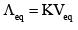Now, G(conductance) = KA/l => K = Gl/A and Veq = Al

So,(Gl/A)(Al) = Gl2

Hence A and B are correct.

*Multiple options can be correct
QUESTION: 11

Choose the correct statements:

Solution:
QUESTION: 12

Choose the correct options:

Solution:

Explanation : molar conductance = Valency × equivalent conductance.

Al2(SO4)3 is commonly known as aluminium sulphate. There is two aluminium atom in aluminium sulphate, and because of this, the total valency of the formula is 6+

AM = 6eq for Al2(SO4)3

*Multiple options can be correct
QUESTION: 13

Which of the following will have same value of molar conductivity and equivalent conductivity:

Solution:
*Multiple options can be correct
QUESTION: 14

If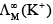= 73.5Ω-1cm2 mol-1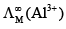= 189Ω-1cm2 mol-1 and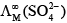= 160Ω-1cm2 mol-1 then:

Solution:

Potash alum is K2SO4 · Al2(SO4)3 · 24H2O
On dilutionsions will be 2K+, 2Al3+ and 4SO42–

M = (2x73.5)+ (2x189) + (4x160)

= 1165 S cm2 mol-1

*Multiple options can be correct
QUESTION: 15

The unit of conductance is (are):

Solution:
*Multiple options can be correct
QUESTION: 16

The unit of conductivity is(are):

Solution:

A and C are correct.

R = ρ l/A

ρ = RA/l

Units of ρ = Ω cm

σ = 1/ρ

Units of σ = Ω-1 cm-1

Ω-1 = S (Siemens)

Units of σ = S cm-1

QUESTION: 17

Choose the correct order of molar ionic conductivities of the following ions.

Solution:
*Multiple options can be correct
QUESTION: 18

For which of the following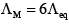relation is correct:

Solution: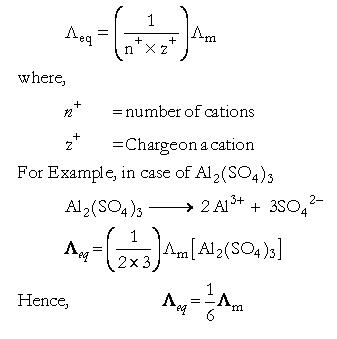*Multiple options can be correct
QUESTION: 19

Choose the correct statements:

Solution:
QUESTION: 20

On dilution, the:

Solution:
*Answer can only contain numeric values
QUESTION: 21

The equivalent conductance of 0.01 M solution of K2SO4 whose specific conductance is 1.26 × 10–3 Ω-1 cm-1 are ________ Ω-1cm2 eq-1

Solution: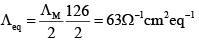*Answer can only contain numeric values
QUESTION: 22

The conductivity of 0.1 M NaOH solution is 0.0221 Ω-1 cm-1. When an equal volume of 0.1 M HCl is added, the conductivity decreases to 0.0056 Ω-1 cm-1. A further addit ion HCl solution, the volume of which of which is equal to that of the first portion added, the conductivity increases to 0.017 Ω-1 cm-1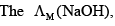Solution: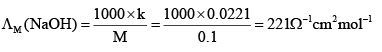*Answer can only contain numeric values
QUESTION: 23

The conductivity of 0.1 M NaOH solution is 0.0221 Ω-1 cm-1. When an equal volume of 0.1 M HCl is added, the conductivity decreases to 0.0056 Ω-1 cm-1. A further addit ion HCl solution, the volume of which of which is equal to that of the first portion added, the conductivity increases to 0.017 Ω-1 cm-1.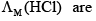________, _____ and _______ Ω-1 cm-1 respectively:

Solution: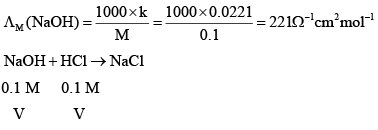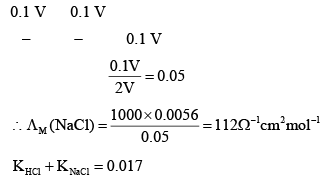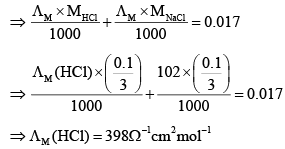*Answer can only contain numeric values
QUESTION: 24

The specific conductance of a saturated solution of AgCl at 25ºC after subtracting the specific conductance of water is 2.28 × 10–4 Sm–1. The molar ionic conductance of Ag+ and Cl ions are 73.3 × 10–4 and 65 × 10–4 Sm2 mol–1. The solubility of AgCl at 25ºC is _______ g/dm3.

Solution: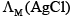= (73.3 + 65) ×10-4 S m2 mol-1 = 0.01383 S m2 mol-1 = 0.01383 ×104 S cm2 mol-1 K = 2.28 ×10-4 S m-1 = 2.28 ×10-6 S cm-1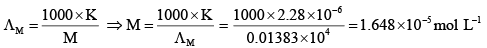1mol of AgCl = 108 + 35.5 = 143.5g
B = 1.648 ×10-5 ×143.5g / L or g / dm3
= 2.36 ×10-3 g dm-3

*Answer can only contain numeric values
QUESTION: 25

The ionic conductivities at infinite dilution of Ox2–, K+ and Na+ are 148.2, 50.1 and 73.5 Ω-1 cm2 mol-1 respectively. The equivalent conductivities at infinite dilution of the salt KOOC. COONa is  _______ Ω-1 cm2 mol-1

Solution: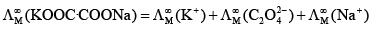= 50.1 + 148.2 + 73.5 = 271.8Ω-1cm 2 mol-1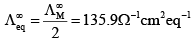*Answer can only contain numeric values
QUESTION: 26

The molar conductivity of NH4Cl at infinite dilution is 149.7 Ω-1 cm2 mol-1 and the ionic conductivities of OH- and Cl- ions are 198 and 76.3 Ω-1 cm2 mol-1 respectively. The molar conductivity of NH4OH at the dilution is _______ Ω-1 cm2 mol-1:

Solution: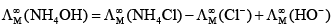= (149.7 - 76.3 + 198)Ω-1cm2 mol-1
= 271.4Ω-1cm2 mol-1

*Answer can only contain numeric values
QUESTION: 27

A conductivity cell whose cell constant is 2cm–1 is filled with 0.1 M acetic acid solution. Its resistance is found to be 3765 Ω. The degree of dissociation of acetic acid are ______ . Given that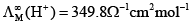and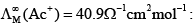Solution: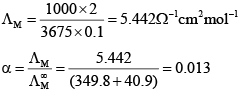*Answer can only contain numeric values
QUESTION: 28

The conductivity of a saturated solution of silver okalate is 4.5 × 10–5 Ω-1 cm-1. If its Ksp = 1.35 × 10-11 M3, the molar conductivity of the saturated solution would be _______  Ω-1 cm2 mol-1.

Solution: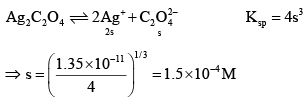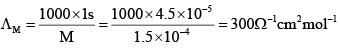*Answer can only contain numeric values
QUESTION: 29

The molar conductivity of 0.05 M of solution of an electrolyte is 200 Ω-1 cm2 mol-1. The resistance offered by a conductivity cell with cell constant (1/3 cm-1) would be _______Ω.

Solution: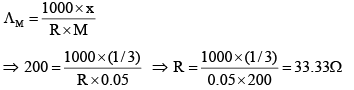*Answer can only contain numeric values
QUESTION: 30

A conductivity cell whose cell constant is 3 cm–1 is filled with 0.1 M solution of weak acids. Its resistance is found to be 3000 Ω.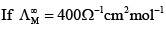the degree of dissociation of weak acid is _______.

Solution: